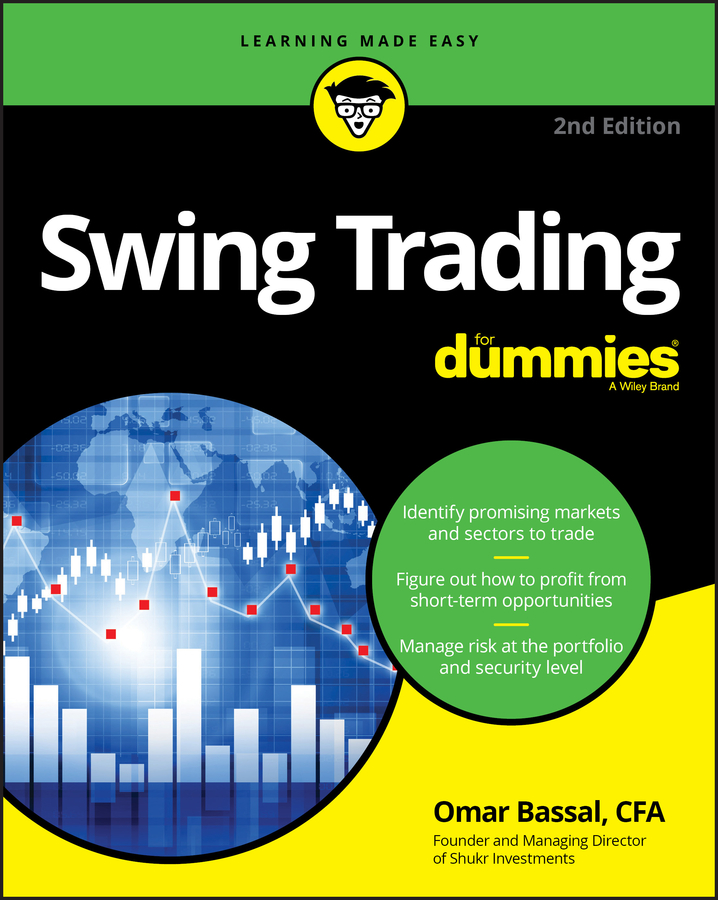##### Swing Trading For DummiesIn practice, security price momentum is really what math nit-pickers call rate of change. It compares the price today with the price x periods ago. A higher number means a faster speed — and momentum is all about speed. The momentum indicator most technical traders use and software packages offer calculates momentum using the rate-of-change method:

1. Divide today’s close by the close a certain number of days ago.

For example, you can look back five days.

2. Multiply that number by 100.

M = (Price Today/Price Five Days Ago) x100

M = (15/10) x 100 = 150

The result is information presented as a ratio (times 100) rather than the simple difference between the two prices. If today’s price is equal to the price five days ago, the centerline now reads 100, meaning that the new price is equal to 100 percent of the price five days ago, which is the same as saying that there is zero change between the two prices.

Imagine that a price is rising at the same pace every day, say \$1, and you’re using a five-day indicator. On Day 6, if you use the rate-of-change division method, the price today is \$16 and the price five days ago was \$11, so your calculation would look like this:

M = (16/11) x 100 = 145.45

It now appears that momentum is moving down. In the subtraction method of calculating momentum section example, you see a flat line at the zero level. In the rate-of-change method, you get a drop from 150 to 145, showing that even though momentum is changing at a constant rate (\$1 per day), it isn’t accelerating.

The momentum indicator can move up or down only if the price is accelerating or decelerating. The momentum indicator can flat line while the price is still moving if the relative pace of change is the same.